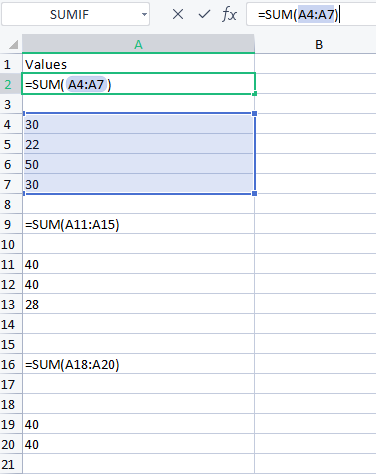# How to calculate the sum by particular rowsPlease provide me the steps and nodes to calculate

Hello @sundipsandy ,

you can achieve this if you have a logical condition for grouping numbers. Like, if numbers in rows from 4 to 7 belong to group “A”, from 11 to 13 to “B” and so on, then sum based on letter will give you the required result.

Alternatively, you can filter or split rows to calculate total sum (ie select only rows from 4 to 7 and then calculate sum, etc etc).

I’d say the first option is the best. Do you have another attribute to make the separation of rows?

Have a nice day,
Raffaello

Hi @lelloba
ThanksBut I need to sum this values in the fixed row. That is row ID’s like 2 ,9 ,16

This isn’t the kind of solution I would normally suggest as it isn’t easy to apply generically, but that said your question isn’t generic either and is dealing with how to get the sum of a group of rows by specific row number rather than by any logical grouping that can be derived programmatically (which is where @lelloba’s suggestion was coming from).

What you are asking in this case can be achieved by three row filters applied separately to your main data set , with each filtering the required rows (by row number, e.g from row 4 to row 7). You can then apply a group by node (calculating sum) to the output from each row filter. That will give you the sums of each range.

It’s not ideal in my view, but it does exactly what you are asking.

I agree with @takbb.

The thing you are trying to do would be totally simple in Excel, but it is much more difficult in Knime, because you work with a different logic.
I’m not saying it is not possible to achieve exactly what you have asked, but maybe changing they way you are going to make the calculation in the first place makes more sense.

Is there a specific reason for which total values are shown in rows 2, 9 and 16? Or is calculating the sum of these groups the only objective?

Thank you,
Raffaello

1 Like

This topic was automatically closed 90 days after the last reply. New replies are no longer allowed.